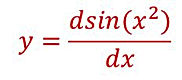naver-site-verification: naver47238a1b6bfbb19a2fd4b619734fa9a6.html
top of page

# Check the analytical derivative or integral on the Ti-84## Check your analytical derivatives or integrals with the TI-84 Plus CE. It is very useful for students in electrical and mechatronics engineering.

With some examples, we will show you how you can check your answers! Let us start by checking the derivate of a function.

Example 1

If you have found the analytical solution and want to check if it is the correct answer, the steps are shown in the following figures.## Check your analytically derived integral with the results of the integral calculated by your Ti-84.

In the same way, you can check your analytical solution of an integral. Suppose next integral with the solution I have derived. The integral which has to be calculated by the Ti84 is substituted in Y1. For the lower limit we choose 0 and the upper limit we choose X instead of a fixed value. Your proposed solution in substituted in Y2. The input for the Ti84 is shown. For the Window range Xmin-Xmax we choose 0-π.The results show (step5) that our derived solution is not correct because Y3 is not equal to zero for the chosen X-range. The error we have made is that we have forgotten the minus sign;  thus the correct solution is :bottom of page